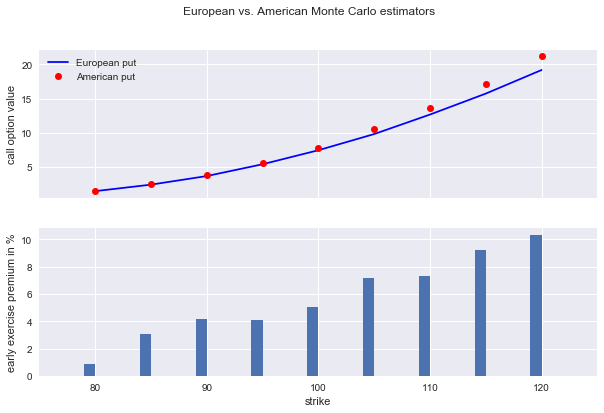# QuantLab: Stochastics¶

### (Go to Quant Lab)¶

#### Source: Python for Finance (2nd ed.)¶

Mastering Data-Driven Finance

© Dr. Yves J. Hilpisch | The Python Quants GmbH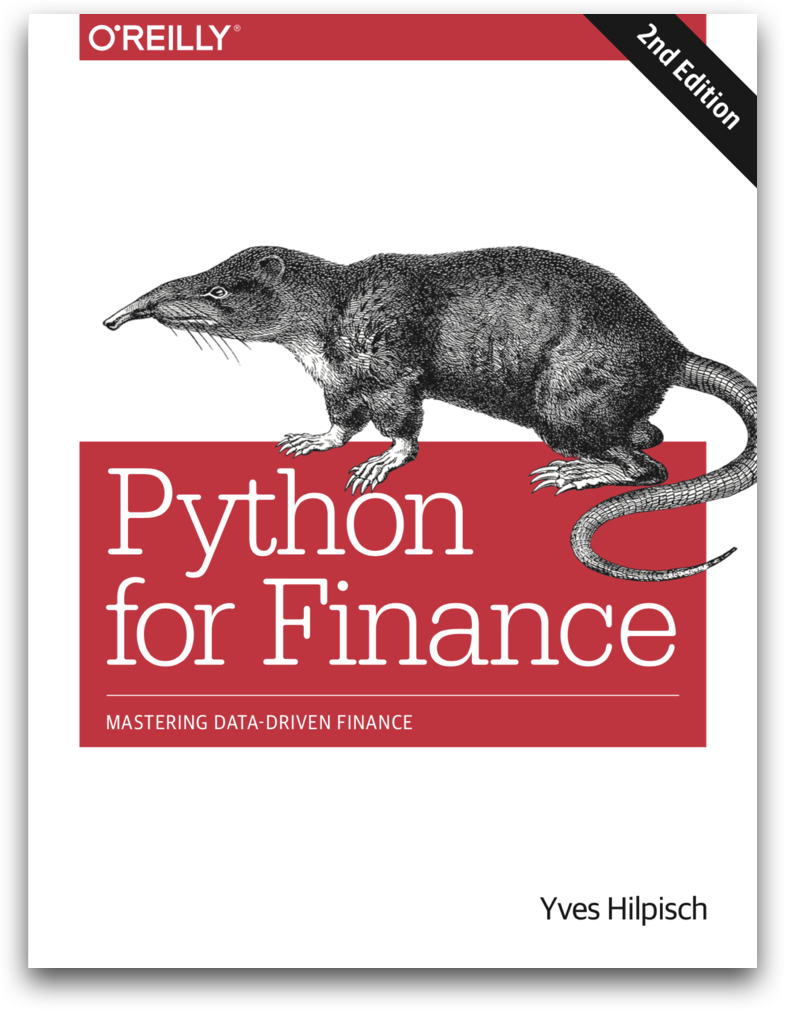Initially import all the modules we will be using for our notebook

In :
import math
import numpy as np
import numpy.random as npr

# COMMAND PROMPT: pip install matplotlib
import matplotlib.pyplot as plt
%matplotlib inline
import os

In :
plt.style.use('seaborn')

In :
# Directory where we will save our plots
directory = "./images"
if not os.path.exists(directory):
os.makedirs(directory)


## 1. Random Numbers¶

First we set the seed in order to always generate the same random numbers.

In :
npr.seed(100)
np.set_printoptions(precision=4)


1.1 Generates $X_1,\ldots\,X_n$ where $X_i \sim U[0,1]$. In this case $n = 10$

In :
npr.rand(10)

Out:
array([0.5434, 0.2784, 0.4245, 0.8448, 0.0047, 0.1216, 0.6707, 0.8259,
0.1367, 0.5751])

1.2 Generates $2$-dimensional $(X,Y)$, where $X_i$ and $Y_j \sim U[0,1]$

In :
npr.rand(5, 5)

Out:
array([[0.8913, 0.2092, 0.1853, 0.1084, 0.2197],
[0.9786, 0.8117, 0.1719, 0.8162, 0.2741],
[0.4317, 0.94  , 0.8176, 0.3361, 0.1754],
[0.3728, 0.0057, 0.2524, 0.7957, 0.0153],
[0.5988, 0.6038, 0.1051, 0.3819, 0.0365]])

1.3 Generates $1$-dimensional $X_1,\ldots\,X_n$ where $X_i \sim U[a,b]$. In other words, we are scaling $U_i \sim U[0,1]$ and adding a drift term, $X_i = a + U_i*(b-a)$

In :
a = 5.
b = 10.
npr.rand(10) * (b - a) + a

Out:
array([9.4521, 9.9046, 5.2997, 9.4527, 7.8845, 8.7124, 8.1509, 7.9092,
5.1022, 6.0501])

1.4 Same transformation as in 1.3) but in $2$ dimensions

In :
npr.rand(5, 5) * (b - a) + a

Out:
array([[7.7234, 8.8456, 6.2535, 6.4295, 9.262 ],
[9.875 , 9.4243, 6.7975, 7.9943, 6.774 ],
[6.701 , 5.8904, 6.1885, 5.2243, 7.5272],
[6.8813, 7.964 , 8.1497, 5.713 , 9.6692],
[9.7319, 8.0115, 6.9388, 6.8159, 6.0217]])

## 2. Plotting Random Samples¶

2.1) First we can compute a couple random samples using random number generators

In :
sample_size = 10000

# Uniformaly distribibuted sample with 3 observations each
rn1 = npr.rand(sample_size, 3)

# Sampling 500 integers between 0-9 (10 is not included)
rn2 = npr.randint(0, 10, sample_size)

# Sampling 500 random floats from [0, 1]
rn3 = npr.sample(size = sample_size)

# Randomly sample values from vector a
a = [0, 25, 50, 75, 100]
rn4 = npr.choice(a, size = sample_size)

In :
# Create 2x2 subplots of each of our random number generators
fig, ((ax1, ax2), (ax3, ax4)) = plt.subplots(nrows=2, ncols=2,
figsize=(10, 8))
ax1.hist(rn1, bins=25, stacked=True)
ax1.set_title('rand')
ax1.set_ylabel('frequency')

ax2.hist(rn2, bins=25)
ax2.set_title('randint')

ax3.hist(rn3, bins=25)
ax3.set_title('sample')
ax3.set_ylabel('frequency')

ax4.hist(rn4, bins=25)
ax4.set_title('choice');

fig.suptitle('Histograms of simple random numbers', fontsize=16)
plt.savefig('./images/stoch_01.png');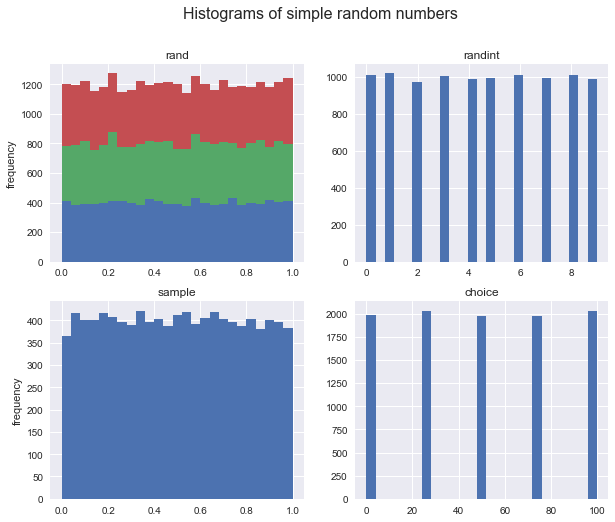2.2) We can also generate randoom numbers from distributions

In :
sample_size = 500

# Generate random samples from standard normal
rn1 = npr.standard_normal(sample_size)

# Generate random samples from normal
rn2 = npr.normal(loc= 100, scale=20, size = sample_size)

# Generate random samples from chisquare
rn3 = npr.chisquare(df= 2, size = sample_size)

# Generate random samples from poisson
rn4 = npr.poisson(lam= 1, size = sample_size)

In :
# Create 2x2 subplots of each of our random number generators
fig, ((ax1, ax2), (ax3, ax4)) = plt.subplots(nrows=2, ncols=2,
figsize=(10, 8))
ax1.hist(rn1, bins=25)
ax1.set_title('standard normal')
ax1.set_ylabel('frequency')

ax2.hist(rn2, bins=25)
ax2.set_title('normal(100, 20)')

ax3.hist(rn3, bins=25)
ax3.set_title('chi square')
ax3.set_ylabel('frequency')

ax4.hist(rn4, bins=25)
ax4.set_title('Poisson');

fig.suptitle('Histograms of random samples for different distributions', fontsize=16)
plt.savefig('./images/stoch_02.png');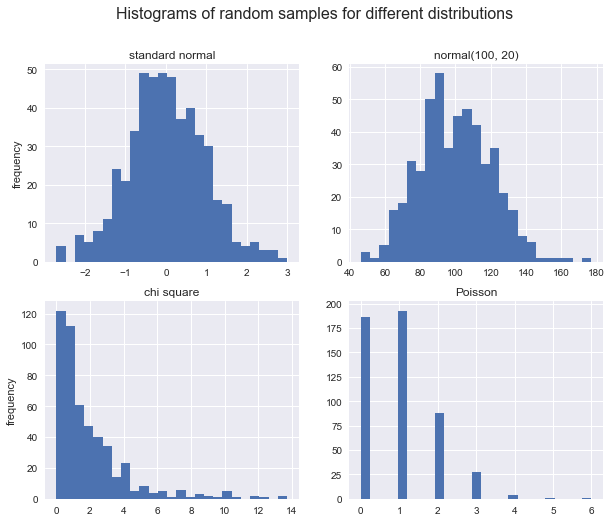## 3. Simulation¶

### 3.1 Random Variables¶

3.1.1) Consider, for example, the Black-Scholes-Merton setup for option pricing. In their setup, the level of a stock index $S_T$ at a future date $T$ given a level $S_0$ as of today is given according to:

$$S_{T}=S_{0}exp\{(r-\frac{1}{2}\sigma^{2})T+\sigma\sqrt{T}z\}$$

where,

• $S_T$ is the index level at date $T$
• $r$ constant riskless short rate
• $\sigma$ constant volatility (standard deviation of returns of $S$)
• $z$ standard normal random variable
In :
S0 = 100
r = 0.05
sigma = 0.25
T = 2.0
I = 10000

ST1 = S0 * np.exp((r - 0.5 * sigma ** 2) * T + sigma * math.sqrt(T) * npr.standard_normal(I))

In :
# Simulation of Geometric Brownian Motion
plt.figure(figsize=(10, 6))
plt.hist(ST1, bins=50)
plt.title('Statically simulated geometric Brownian motion (via npr.standard_normal())')
plt.xlabel('index level')
plt.ylabel('frequency');
plt.savefig('./images/stoch_03.png');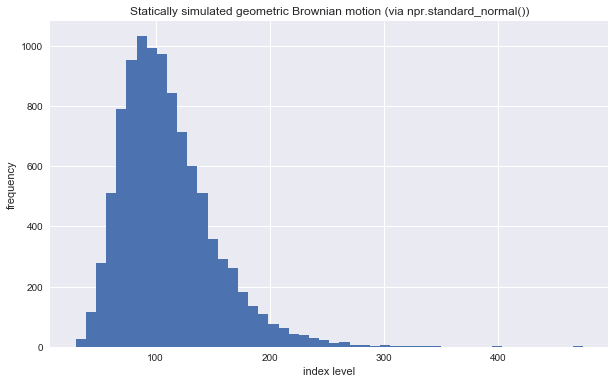3.1.2) Alternatively since we know that the equation in 3.1.1) represents a log-normal distribution, we can use the numpy's lognormal random generator by specifying location and scale parameters.

Recall by definition of log-normal, \begin{align*} \mu & =(r-\frac{1}{2}\sigma^{2})T\\ \sigma & =\sigma\sqrt{T} \end{align*}

In :
ST2 = S0 * npr.lognormal(mean = (r - 0.5 * sigma ** 2) * T, sigma = sigma * math.sqrt(T), size= I)

In :
plt.figure(figsize=(10, 6))
plt.hist(ST2, bins=50)
plt.title('Statically simulated geometric Brownian motion (via npr.lognormal())')
plt.xlabel('index level')
plt.ylabel('frequency');
plt.savefig('./images/stoch_04.png');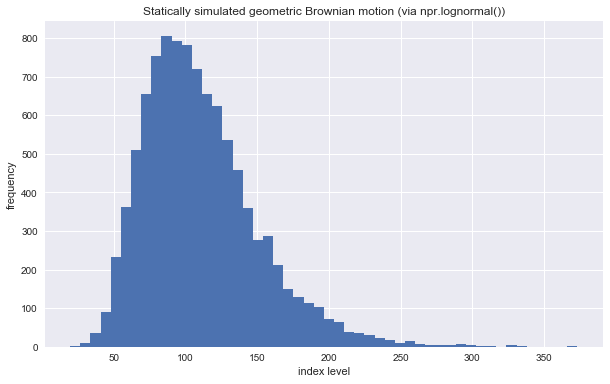3.1.3) In order to conclude that the two simulations above are indeed the same, we can compute some statistics on the two distributions and compare them.

In :
import scipy.stats as scs

In :
def print_statistics(a1, a2):
''' Prints selected statistics.

Parameters
==========
a1, a2: ndarray objects
results objects from simulation
'''
sta1 = scs.describe(a1)
sta2 = scs.describe(a2)
print('%14s %14s %14s' %
('statistic', 'data set 1', 'data set 2'))
print(45 * "-")
print('%14s %14.3f %14.3f' % ('size', sta1, sta2))
print('%14s %14.3f %14.3f' % ('min', sta1, sta2))
print('%14s %14.3f %14.3f' % ('max', sta1, sta2))
print('%14s %14.3f %14.3f' % ('mean', sta1, sta2))
print('%14s %14.3f %14.3f' % ('std', np.sqrt(sta1), np.sqrt(sta2)))
print('%14s %14.3f %14.3f' % ('skew', sta1, sta2))
print('%14s %14.3f %14.3f' % ('kurtosis', sta1, sta2))

In :
print_statistics(ST1, ST2)

     statistic     data set 1     data set 2
---------------------------------------------
size      10000.000      10000.000
min         32.327         28.230
max        414.825        409.110
mean        110.730        110.431
std         40.300         39.878
skew          1.122          1.115
kurtosis          2.438          2.217


### 3.2 Stochastic Processes¶

#### 3.2.1 Geometric Brownian Motion¶

Below we have the Stochastic differential equation in Black-Scholes-Merton:

$$dS_{t}=rS_{t}dt+\sigma S_{t}dZ_{t},$$

which can be discretized exactly by an Euler scheme, $$S_{t}=S_{t-\Delta t}exp\{(r-\frac{1}{2}\sigma^{2})\Delta t+\sigma\sqrt{\Delta t}z_{t}\}$$ Thus translating into python,

In :
S0 = 100      # initial value
I = 10000     # number of paths to simulate
sigma = 0.25
M = 50        # number of time intervals
dt = T / M    # length of time interval in fractions of a year
S = np.zeros(shape= (M + 1, I))  # 2-dim array to store index levels
S = S0     # initializing first row of array with initial value when t = 0

# The for loop provides 10,000 different paths for each time step depending
# on the previous time step. Note this is done in a vectorized way
for t in range(1, M + 1):
S[t] = S[t - 1] * np.exp((r - 0.5 * sigma ** 2) * dt +
sigma * math.sqrt(dt) * npr.standard_normal(I))

In :
# The last array of array S represents all simulated values for S_T
plt.figure(figsize=(10, 6))
plt.hist(S[-1], bins=50)
plt.title('Dynamically simulated geometric Brownian motion at maturity')
plt.xlabel('index level')
plt.ylabel('frequency');
plt.savefig('./images/stoch_05.png');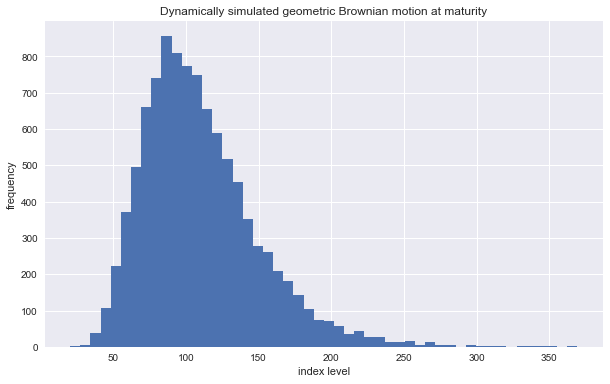Following is a comparison of the statistics resulting from the dynamic simulation as well as from the static simulation.

In :
print_statistics(S[-1], ST2)

     statistic     data set 1     data set 2
---------------------------------------------
size      10000.000      10000.000
min         20.265         19.718
max        369.431        373.244
mean        109.811        110.696
std         39.750         40.142
skew          1.146          1.095
kurtosis          2.353          2.120


Also the figure below shows the first 10 simulated paths:

In :
plt.figure(figsize=(10, 6))
plt.plot(S[:, :10], lw=1.5)
plt.title('Dynamically simulated geometric Brownian motion paths')
plt.xlabel('time')
plt.ylabel('index level');
plt.savefig('./images/stoch_06.png');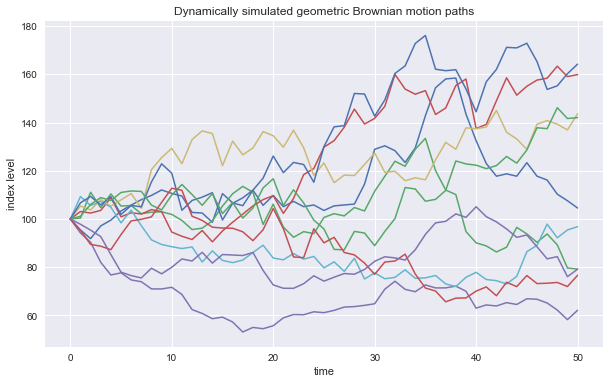Using the dynamic simulation approach not only allows us to visualize paths as displayed in the figure above, but also to value options with American/Bermudan exercise or options whose payoff is path-dependent.

## 4. Valuation¶

### 4.1 Valuation of European call options in Black-Scholes-Merton model¶

In :
def bsm_call_value(S0, K, T, r, sigma):
''' Valuation of European call option in BSM model.
Analytical formula.

Parameters
==========
S0: float
initial stock/index level
K: float
strike price
T: float
maturity date (in year fractions)
r: float
constant risk-free short rate
sigma: float
volatility factor in diffusion term

Returns
=======
value: float
present value of the European call option
'''
from math import log, sqrt, exp
from scipy import stats

S0 = float(S0)
d1 = (log(S0 / K) + (r + 0.5 * sigma ** 2) * T) / (sigma * sqrt(T))
d2 = (log(S0 / K) + (r - 0.5 * sigma ** 2) * T) / (sigma * sqrt(T))
# stats.norm.cdf --> cumulative distribution function
#                    for normal distribution
value = (S0 * stats.norm.cdf(d1, 0.0, 1.0) -
K * exp(-r * T) * stats.norm.cdf(d2, 0.0, 1.0))
return value


### 4.2 Vega function and implied volatility estimation¶

In :
def bsm_vega(S0, K, T, r, sigma):
''' Vega of European option in BSM model.

Parameters
==========
S0: float
initial stock/index level
K: float
strike price
T: float
maturity date (in year fractions)
r: float
constant risk-free short rate
sigma: float
volatility factor in diffusion term

Returns
=======
vega: float
partial derivative of BSM formula with respect
to sigma, i.e. Vega

'''
from math import log, sqrt
from scipy import stats

S0 = float(S0)
d1 = (log(S0 / K) + (r + 0.5 * sigma ** 2) * T) / (sigma * sqrt(T))
vega = S0 * stats.norm.pdf(d1, 0.0, 1.0) * sqrt(T)
return vega

def bsm_call_imp_vol(S0, K, T, r, C0, sigma_est, it=100):
''' Implied volatility of European call option in BSM model.

Parameters
==========
S0: float
initial stock/index level
K: float
strike price
T: float
maturity date (in year fractions)
r: float
constant risk-free short rate
sigma_est: float
estimate of impl. volatility
it: integer
number of iterations

Returns
=======
simga_est: float
numerically estimated implied volatility
'''
for i in range(it):
sigma_est -= ((bsm_call_value(S0, K, T, r, sigma_est) - C0) /
bsm_vega(S0, K, T, r, sigma_est))
return sigma_est


### 4.3 Pricing European Options by Monte Carlo¶

The payoff of a European call option on an index at maturity is given by $h(S_T)\equiv max\{S_T-K, 0\}$, where $S_T$ is the index level at maturity date $T$ and $K$ is the strike price. Given a risk-neutral measure for the relevant stochastic process (e.g., geometric Brownian motion), the price of such an option is given by the formula:

$$C_{0}=e^{-rT}\mathbb{E}_{0}^{Q}[h(S_{T})]=e^{-rT}\intop_{0}^{\infty}h(s)q(s)ds$$

The equation below provides the respective Monte Carlo estimator for the European option, where $\tilde{S}_{T}^{i}$ is the $T$-th simulated index level at maturity.

$$\tilde{C}_{0}=e^{-rT}\frac{1}{I}\sum_{i=1}^{I}h(\tilde{S}_{T}^{i})$$

Consider the following parameterization for the geometric Brownian motion and the valuation function gbm_mcs_stat(), taking as a parameter only the strike price. Here, only the index level at maturity is simulated. As a reference, consider the case with a strike price of $K = 105$:

In :
def gen_sn(M, I, anti_paths=True, mo_match=True):
''' Function to generate random numbers for simulation.

Parameters
==========
M: int
number of time intervals for discretization
I: int
number of paths to be simulated
anti_paths: boolean
use of antithetic variates
mo_math: boolean
use of moment matching
'''
if anti_paths is True:
sn = npr.standard_normal((M + 1, int(I / 2)))
sn = np.concatenate((sn, -sn), axis=1)
else:
sn = npr.standard_normal((M + 1, I))
if mo_match is True:
sn = (sn - sn.mean()) / sn.std()
return sn

In :
S0 = 100.
r = 0.05
sigma = 0.25
T = 1.0
I = 50000

In :
def gbm_mcs_stat(K):
''' Valuation of European call option in Black-Scholes-Merton
by Monte Carlo simulation (of index level at maturity)

Parameters
==========
K: float
(positive) strike price of the option

Returns
=======
C0: float
estimated present value of European call option
'''
sn = gen_sn(1, I)
# simulate index level at maturity
ST = S0 * np.exp((r - 0.5 * sigma ** 2) * T
+ sigma * math.sqrt(T) * sn)
# calculate payoff at maturity
hT = np.maximum(ST - K, 0)
# calculate MCS estimator
C0 = math.exp(-r * T) * np.mean(hT)
return C0

In :
# The Monte Carlo estimator value for the European call option.
gbm_mcs_stat(K=105.)

Out:
10.010189184997577

Next, consider the dynamic simulation approach and allow for European put options in addition to the call option. The function gbm_mcs_dyna() implements the algorithm. The code also compares option price estimates for a call and a put stroke at the same level:

In :
# The number of time intervals for the discretization.
M = 50

In :
def gbm_mcs_dyna(K, option='call'):
''' Valuation of European options in Black-Scholes-Merton
by Monte Carlo simulation (of index level paths)

Parameters
==========
K: float
(positive) strike price of the option
option : string
type of the option to be valued ('call', 'put')

Returns
=======
C0: float
estimated present value of European call option
'''
dt = T / M

# simulation of index level paths
S = np.zeros((M + 1, I))
S = S0
sn = gen_sn(M, I)

for t in range(1, M + 1):
S[t] = S[t - 1] * np.exp((r - 0.5 * sigma ** 2) * dt
+ sigma * math.sqrt(dt) * sn[t])

# case-based calculation of payoff
if option == 'call':
hT = np.maximum(S[-1] - K, 0)
else:
# Put option
hT = np.maximum(K - S[-1], 0)
# calculation of MCS estimator
C0 = math.exp(-r * T) * np.mean(hT)
return C0

In :
# The Monte Carlo estimator value for the European call option (Dynamic Simulation Approach)
gbm_mcs_dyna(K=110., option='call')

Out:
7.987266071170579
In :
# The Monte Carlo estimator value for the European put option (Dynamic Simulation Approach)
gbm_mcs_dyna(K=110., option='put')

Out:
12.623515262341943

The question is how well these simulation-based valuation approaches perform relative to the benchmark value from the Black-Scholes-Merton valuation formula. To find out, the following code generates respective option values/estimates for a range of strike prices, using the analytical option pricing formula for European calls. First, we compare the results from the static simulation approach with precise analytical values:

In :
# Instantiates empty list objects to collect the results.
stat_res = []
dyna_res = []
anal_res = []

# Creates an ndarray object containing the range of strike prices.
k_list = np.arange(80., 120.1, 5.)
np.random.seed(100)

In :
# Simulates/calculates and collects the option values for all strike prices.
for K in k_list:
stat_res.append(gbm_mcs_stat(K))
dyna_res.append(gbm_mcs_dyna(K))
anal_res.append(bsm_call_value(S0, K, T, r, sigma))

In :
# Transforms the list objects to ndarray objects.
stat_res = np.array(stat_res)
dyna_res = np.array(dyna_res)
anal_res = np.array(anal_res)


The figure below shows the results. All valuation differences are smaller than 1% absolutely. There are both negative and positive value differences:

In :
plt.figure(figsize=(10, 6))
fig, (ax1, ax2) = plt.subplots(2, 1, sharex=True, figsize=(10, 6))
ax1.plot(k_list, anal_res, 'b', label='analytical')
ax1.plot(k_list, stat_res, 'ro', label='static')
ax1.set_ylabel('European call option value')
ax1.legend(loc=0)
ax1.set_ylim(bottom=0)
wi = 1.0
ax2.bar(k_list - wi / 2, (anal_res - stat_res) / anal_res * 100, wi)
ax2.set_xlabel('strike')
ax2.set_ylabel('difference in %')
ax2.set_xlim(left=75, right=125);
fig.suptitle('Analytical option values vs. Monte Carlo estimators (static simulation)')
plt.savefig('./images/stoch_15.png');

<Figure size 720x432 with 0 Axes>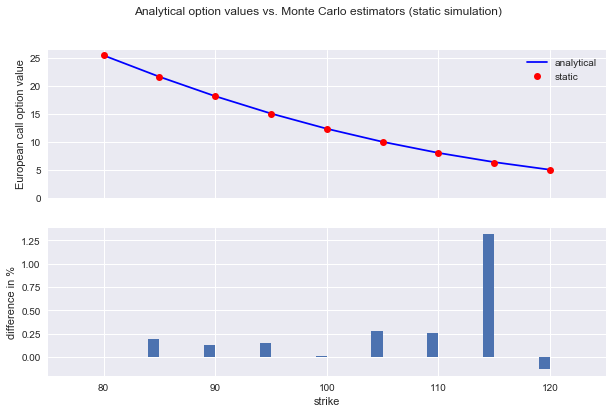A similar picture emerges for the dynamic simulation and valuation approach, whose results are reported in the figure below. Again, all valuation differences are smaller than 1% absolutely, with both positive and negative deviations. As a general rule, the quality of the Monte Carlo estimator can be controlled for by adjusting the number of time intervals $M$ used and/or the number of paths $I$ simulated:

In :
fig, (ax1, ax2) = plt.subplots(2, 1, sharex=True, figsize=(10, 6))
ax1.plot(k_list, anal_res, 'b', label='analytical')
ax1.plot(k_list, dyna_res, 'ro', label='dynamic')
ax1.set_ylabel('European call option value')
ax1.legend(loc=0)
ax1.set_ylim(bottom=0)
wi = 1.0
ax2.bar(k_list - wi / 2, (anal_res - dyna_res) / anal_res * 100, wi)
ax2.set_xlabel('strike')
ax2.set_ylabel('difference in %')
ax2.set_xlim(left=75, right=125);
fig.suptitle('Analytical option values vs. Monte Carlo estimators (dynamic simulation)')
plt.savefig('./images/stoch_16.png');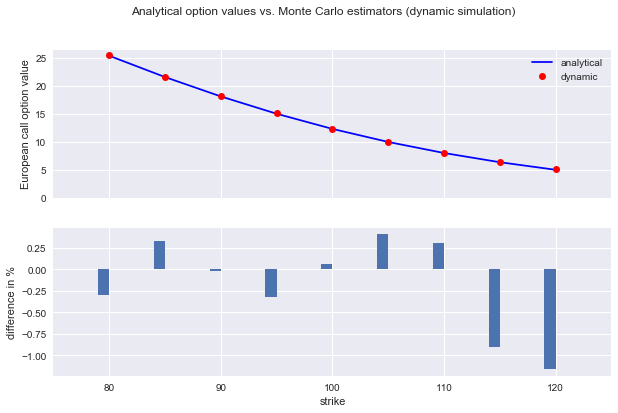### 4.4 American Options¶

The valuation of American options is more involved compared to European options. In this case, an optimal stopping problem has to be solved to come up with a fair value of the option. The Equation below formulates the valuation of an American option as such a problem. The problem formulation is already based on a discrete time grid for use with numerical simulation. In a sense, it is therefore more correct to speak of an option value given Bermudan exercise. For the time interval converging to zero length, the value of the Bermudan option converges to the one of the American option.

$$V_{0}=\sup_{\tau\in\{0,\Delta t,2\Delta t,\dots,T\}}e^{-rT}\mathbb{E}_{0}^{Q}[h_{\tau}(S_{\tau})]$$

The algorithm described in the following is called Least-Squares Monte Carlo (LSM) and is from the paper by Longstaff and Schwartz (2001). It can be shown that the value of an American (Bermudan) option at any given date $t$ is given as

$$V_t(s)=max\{h_t(s),C_t(s)\}$$

, where

$$C_{t}(s)=\mathbb{E}_{t}^{Q}[e^{-r\Delta t}V_{t+\Delta t}(S_{t+\Delta t})|S_{t}=s]$$

is the so-called continuation value of the option given an index level of $S_t=s$

Consider now that we have simulated $I$ paths of the index level over $M$ time intervals of equal size $\Delta t$.

Define $Y_{t,i}\equiv e^{-r\Delta t}V_{t+\Delta t,i}$ to be the simulated continuation value for path $i$ at time $t$. We cannot use this number directly because it would imply perfect foresight. However, we can use the cross section of all such simulated continuation values to estimate the (expected) continuation value by least-squares regression.

Given a set of basis functions $b_d,d=1,\dots,D$, the continuation value is then given by the regression estimate $\hat{C}_{t,i}=\sum_{d=1}^{D}\alpha_{d,t}^{*}\cdot b_{d}(S_{t,i})$, where the optimal regression parameters $\alpha^*$ are the solution of the least-squares problem stated in the equation below:

$$\min_{\alpha_{1,t},\dots,\alpha_{D,t}}\frac{1}{I}\sum_{i=1}^{I}(Y_{t,i}-\sum_{d=1}^{D}\alpha_{d,t}\cdot b_{d}(S_{t,i}))^{2}$$
In :
# The function gbm_mcs_amer() implements the LSM algorithm for both American call and put options
def gbm_mcs_amer(K, option='call'):
''' Valuation of American option in Black-Scholes-Merton
by Monte Carlo simulation by LSM algorithm

Parameters
==========
K : float
(positive) strike price of the option
option : string
type of the option to be valued ('call', 'put')

Returns
=======
C0 : float
estimated present value of European call option
'''
dt = T / M
df = math.exp(-r * dt)
# simulation of index levels
S = np.zeros((M + 1, I))
S = S0
sn = gen_sn(M, I)
for t in range(1, M + 1):
S[t] = S[t - 1] * np.exp((r - 0.5 * sigma ** 2) * dt
+ sigma * math.sqrt(dt) * sn[t])
# case based calculation of payoff
if option == 'call':
h = np.maximum(S - K, 0)
else:
h = np.maximum(K - S, 0)
# LSM algorithm
V = np.copy(h)
for t in range(M - 1, 0, -1):
reg = np.polyfit(S[t], V[t + 1] * df, 7)
C = np.polyval(reg, S[t])
V[t] = np.where(C > h[t], V[t + 1] * df, h[t])
# MCS estimator
C0 = df * np.mean(V)
return C0

In :
gbm_mcs_amer(110., option='call')

Out:
7.721705606305352
In :
gbm_mcs_amer(110., option='put')

Out:
13.609997625418051

The European value of an option represents a lower bound to the American option’s value. The difference is generally called the early exercise premium. What follows compares European and American option values for the same range of strikes as before to estimate the early exercise premium, this time with puts:

In :
euro_res = []
amer_res = []

In :
k_list = np.arange(80., 120.1, 5.)

In :
for K in k_list:
euro_res.append(gbm_mcs_dyna(K, 'put'))
amer_res.append(gbm_mcs_amer(K, 'put'))

In :
euro_res = np.array(euro_res)
amer_res = np.array(amer_res)


The figure below shows that for the range of strikes chosen the early exercise premium can rise to up to 10%:

In :
fig, (ax1, ax2) = plt.subplots(2, 1, sharex=True, figsize=(10, 6))
ax1.plot(k_list, euro_res, 'b', label='European put')
ax1.plot(k_list, amer_res, 'ro', label='American put')
ax1.set_ylabel('call option value')
ax1.legend(loc=0)
wi = 1.0
ax2.bar(k_list - wi / 2, (amer_res - euro_res) / euro_res * 100, wi)
ax2.set_xlabel('strike')